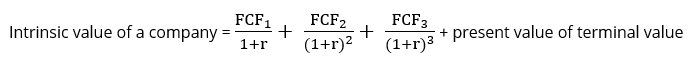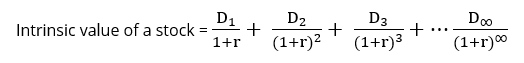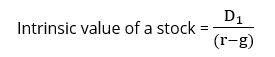## What is Intrinsic Valuation?

Intrinsic or absolute valuation is a method of valuing a business based on the present value of its future cash flows. It relies on the valuer’s expectations of how the business will evolve, including its growth rate, margins, and investment levels. Unlike market-based valuation methodologies, it does not rely on the market price of comparable companies operating in a similar industry as the basis of its valuation. Intrinsic valuation can be used to value both public and private companies.

There are two methods used for calculating intrinsic valuation: calculating the value of the company’s operations (the discounted cash flow method) or calculating the value of a company’s stock (the dividend discount model).

These valuation techniques are often used in conjunction with market-based valuation techniques to act as a comparison and sense check. However, they also offer important insight into companies where there are “few” or no comparable businesses, due to differences in business models or growth expectations. This is particularly the case when valuing young, high growth companies which seek to disrupt the competition.

## Key Learning Points

• Intrinsic valuation is a valuation technique that uses company data and assumptions to value a business
• It is best practice to value a business using both intrinsic and relative valuation methodologies as these act as a comparison and sense check
• There are two intrinsic valuation methods: a discounted cash flow (DCF) or dividend discount model
• A DCF analysis involves discounting a company’s future cash flows to today’s value known as the enterprise value
• The dividend discount model values a company based on its present value of future dividends

## Discounted Cash Flow Method

The discounted cash flow (DCF) method calculates the value of a company’s operations, which is also referred to as its enterprise value. The DCF method calculates the enterprise value as the present value of a company’s future cash flows. Since companies are assumed to continue trading indefinitely, the value reflects cash flows generated from today into perpetuity.

The DCF method, therefore, requires us to forecast a company’s cash flows. As we are valuing the company’s operations, the cash flows used in the calculation are those generated by the operations and available to all providers of finance. They are therefore also referred to as the firm’s free cash flows or unlevered free cash flows.

The forecast cash flows from today until perpetuity, are then discounted to “today’s” value, using the following formula:Where,

FCFn = Expected free cash flow for each period

r = Average rate of return expected by debt and equity investors

Clearly, it is not possible to explicitly forecast cash flows for every year into perpetuity.  Therefore, in practice, we typically forecast the cash flows of a company for 3-10 years (or until the company reaches a stable growth rate). The cash flows beyond our explicit forecast period are then assumed to grow at a constant growth rate each year. The value of the cash flows beyond our explicit forecast period is referred to as the terminal value.

We can therefore replace the formula above with:## Dividend Discount Model (DDM)

In the DDM method, a stock is valued based on the present value of future dividends. This method still relies on the premise that value is based on the present value of future cash flows but, because it values equity – rather than the operations – the cash flows used are only those to equity investors i.e. dividends. This technique is most widely used when valuing financial companies (such as banks and insurance companies) as it can be difficult to separate the operations of these businesses from the financing of them.

We can show the intrinsic value of a stock using the following formula:Where

D1 = Expected dividend for each period

r = Rate of return expected by equity investors

An example of the DDM is the Gordon Growth Model. For this model, we assume that dividends will always grow at a constant growth rate. The formula for the Gordon Growth Model is:Where

D1 = Expected dividend for the next period

r = Rate of return expected by equity investors

g = Growth rate for dividends, into perpetuity

Let us say a company is expected to pay a dividend of 2.0 next year for each common share. The expected rate of return is 5.0% and the growth rate for dividends is 2.0%. How much is the intrinsic value of this stock?

Intrinsic value = 2.0/(5.0%-2.0%) = 66.7